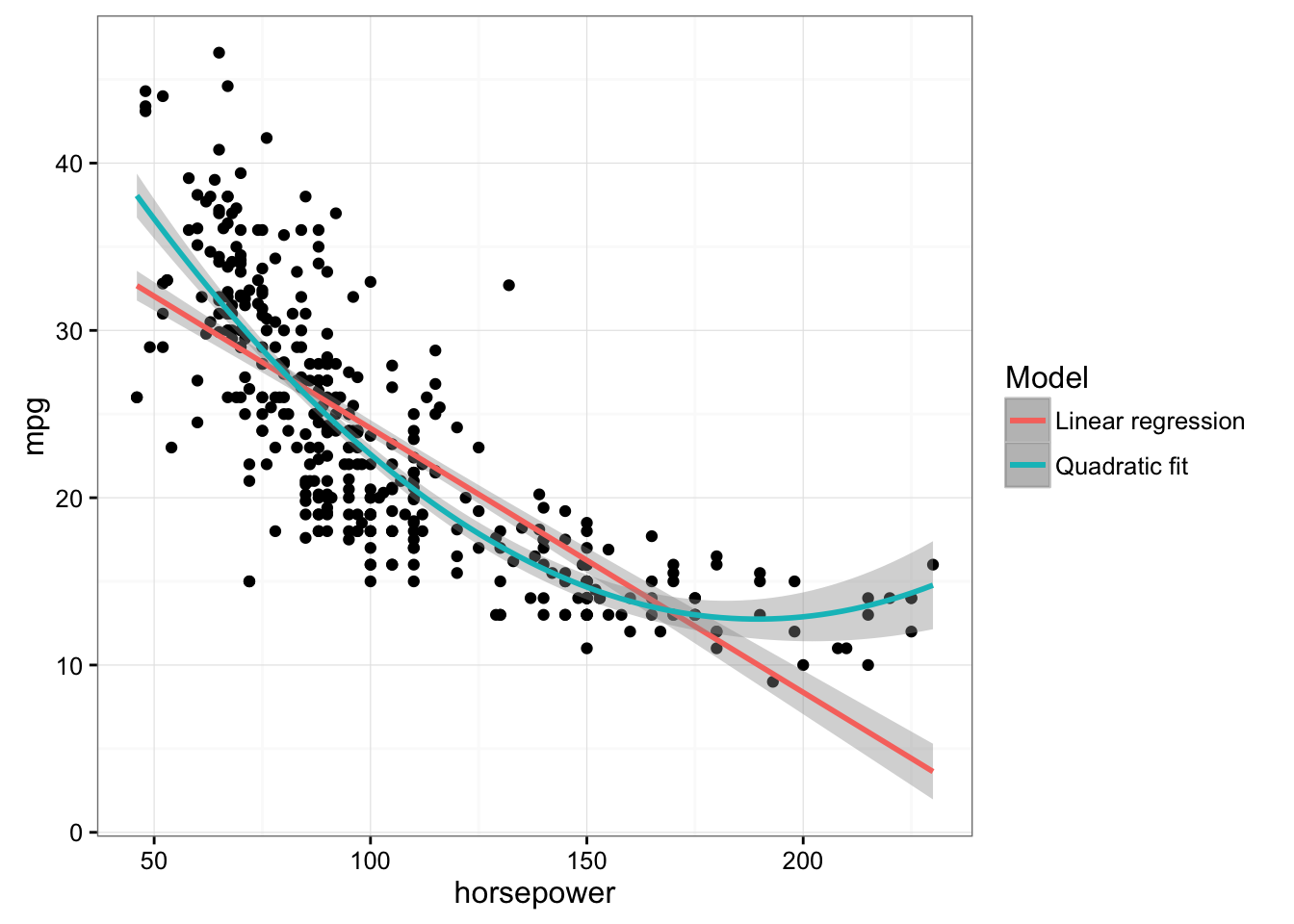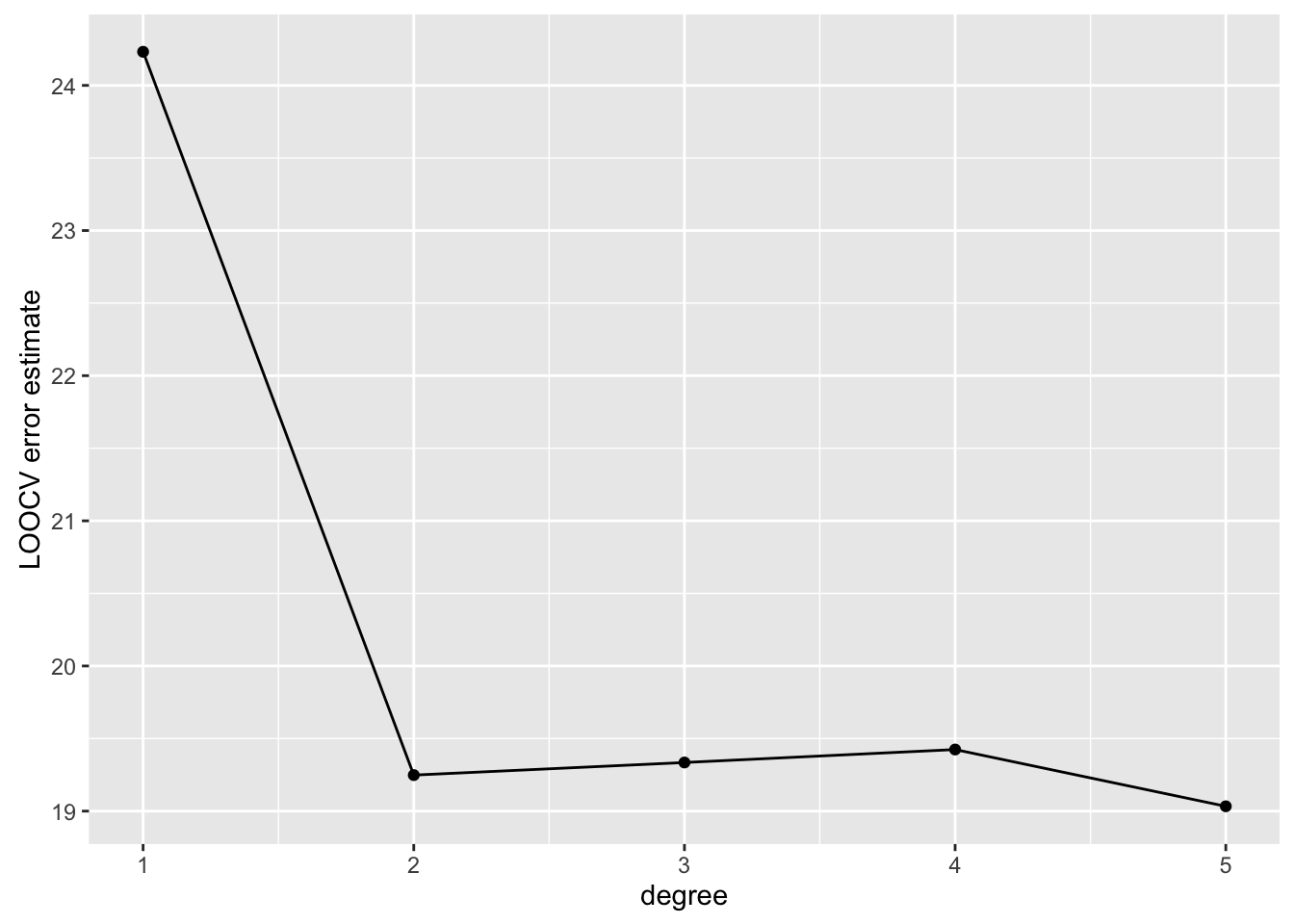``````library(ggplot2) # graphics library
library(MASS)    # contains data sets
library(ISLR)    # contains code and data from the textbook
library(knitr)   # contains kable() function
library(boot)    # contains cross-validation functions
library(gam)     # needed for additive models``````
``## Loading required package: splines``
``## Loading required package: foreach``
``## Loaded gam 1.14``
``options(scipen = 4)  # Suppresses scientific notation``

### 1. Changing the author field and file name.

##### (b) Rename this file to “lab01_YourHameHere.Rmd”, where YourNameHere is changed to your own name.

The next portion of the lab gets you to carry out the Lab in §5.3 of ISLR (Pages 191 - 193). You will want to have the textbook Lab open in front you as you go through these exercises. The ISLR Lab provides much more context and explanation for what you’re doing.

### 2. The Validtion Set Approach

You will need the `Auto` data set from the `ISLR` library in order to complete this exercise.

Please run all of the code indicated in §5.3.1 of ISLR, even if I don’t explicitly ask you to do so in this document.

##### (a) Run the `View()` command on the `Auto` data to see what the data set looks like.
``#View(Auto)``
##### Use `qplot` to construct a scatterplot of `mpg` vs `horsepower`. Use `stat_smooth()` to overlay a linear, quadratic, and cubic polynomial fit to the data.
``````qplot(data = Auto, x = horsepower, y = mpg) +
stat_smooth(method = "lm", aes(colour = "Linear regression")) +
stat_smooth(method = "lm", formula = y ~ poly(x, 2), aes(colour = "Quadratic fit")) +
scale_colour_discrete("Model") +
theme_bw()``````##### (b) Use the command `set.seed(1)` to set the seed of the random number generator. This will ensure that your answers match those in the text.
``````# Edit me
set.seed(1)``````
##### (c) Use the `sample()` command to construct `train`, a vector of observation indexes to be used for the purpose of training your model.
``````# Edit me
train <- sample(392, 196)``````
##### Describe what the sample() function as used above actually does.
• When used in `sample(n, size)` syntax, the `sample` function produces a random sample of size `size` from `1:n`. Sampling is done without replacement.
##### (c) Fit a linear model regression `mpg` on `horsepower`, specifying `subset = train`. Save this in a variable called `lm.fit`.
``````# Edit me
lm.fit <- lm(mpg ~ horsepower, data = Auto, subset = train) ``````
##### Why do we use the argument `subset = train`?
• The `train` indexes form the training set. We want to train on just the `train` data, not the full data.
##### (d) Calculate the MSE of `lm.fit` on the test set (i.e., all points that are not in `train`)
``````mse1 <- with(Auto, mean((mpg - predict(lm.fit, Auto))[-train]^2))
mse1``````
``##  26.14142``
##### (e) Use the `poly()` command to fit a quadratic regression model of `mpg` on `horsepower`, specifying `subset = train`. Save this in a variable called `lm.fit2`.
``lm.fit2 <- lm(mpg ~ poly(horsepower, 2), data = Auto, subset = train)``
##### Is the coefficient of the quadratic term statistically significant?
``````# Pull the p-value
coef(summary(lm.fit2))["poly(horsepower, 2)2", "Pr(>|t|)"]``````
``##  6.449276e-09``
• Yes, the coefficient is highly statistically significant
##### Calculate the test MSE of `lm.fit2`. How does it compare to the linear regression fit?
``````mse2 <- with(Auto, mean((mpg - predict(lm.fit2, Auto))[-train]^2))
mse2``````
``##  19.82259``
• The test error of the quadratic model is 19.8, which is considerably lower than the test error of the linear model 26.1.
##### (f) Use the `poly()` command to fit a cubic regression model of `mpg` on `horsepower`, specifying `subset = train`. Save this in a variable called `lm.fit3`.
``lm.fit3 <- lm(mpg ~ poly(horsepower, 3), data = Auto, subset = train)``
##### Is the coefficient of the cubic term statistically significant?
``````# Pull the p-value
coef(summary(lm.fit3))["poly(horsepower, 3)3", "Pr(>|t|)"]``````
``##  0.6791491``
• No, the coefficient of the cubic term is not statistically significant. It looks like going from quadratic to cubic doesn’t help to explain much additional variability in the data
##### Calculate the test error of the cubic fit.
``````mse3 <- with(Auto, mean((mpg - predict(lm.fit3, Auto))[-train]^2))
mse3``````
``##  19.78252``
##### (g) How do the test errors of the three models compare? Which of the three models should we use?
``mse1 # Linear test error``
``##  26.14142``
``mse2 # Quadratic test error``
``##  19.82259``
``mse3 # Cubic test error``
``##  19.78252``
• While the cubic model has ever-so-slightly lower test error, it’s very close to the test error of the quadratic model. Given that the cubic term isn’t even statistically significant, the quadratic model is the best model to use. The quadratic does much better than the linear model.
##### What does changing the seed value do? Did this change your estimated test errors? Why did the values change? Should we still pick the same model?
• When you re-run the code with `set.seed(5)`, you are getting a different random split of the data between Train and Validation. The estimates of test error also change, because we now have slightly different model estimates, and a different random set to test the models on. We now get an error of `22.2` for the linear model; `15.2` for the quadratic, and `15.2` for the cubic. These numbers are quite different from our estimates based on the first split we tried. However, the conclusion is essentially the same: The cubic model doesn’t seem to add much, but the quadratic is much better than the linear. We should use the quadratic model.

### 3. Leave-One-Out Cross-Validation

This exercise introduces you to the `cv.glm()` command from the `boot` library, which automates K-fold cross-validation for Generalized Linear Models (GLMs). Linear regression is one example of a GLM. Logistic regression is another. GLMs are not the same as Generalized Additive Models (GAMs).

Please run all of the code indicated in §5.3.2 of ISLR, even if I don’t explicitly ask you to do so in this document.

##### (a) Use the `glm` command to fit a linear regression of `mpg` on `horsepower`. Call the resulting model `glm.fit` Confirm that this gives the same coefficient estimates as a linear model fit with the `lm` command.

Note: You should fit the model to the entire data, not just to the training data.

``````glm.fit <- glm(mpg ~ horsepower, data = Auto)

coef(glm.fit)``````
``````## (Intercept)  horsepower
##  39.9358610  -0.1578447``````
``coef(lm(mpg ~ horsepower, data = Auto))``
``````## (Intercept)  horsepower
##  39.9358610  -0.1578447``````
``````# Are they the same? Yes.
identical(coef(glm.fit), coef(lm(mpg ~ horsepower, data = Auto)))``````
``##  TRUE``
##### (b) Run all of the code in §5.3.2. Construct a plot of `cv.error`, the vector of LOOCV error estimates for polynomials of degree 1-5.

Note: The computations take some time to run, so I’ve set `cache = TRUE` in the code chunk header to make sure that the code below doesn’t re-execute at knit time unless this particular chunk has changed.

``````cv.err <- cv.glm(Auto, glm.fit)
cv.err\$delta``````
``##  24.23151 24.23114``
``````cv.error <- rep(0, 5)
for (i in 1:5) {
glm.fit <- glm(mpg ~ poly(horsepower, i), data=Auto)
cv.error[i] <- cv.glm(Auto, glm.fit)\$delta
}
cv.error``````
``##  24.23151 19.24821 19.33498 19.42443 19.03321``
``````# Form a little data frame for plotting
cv.df <- data.frame(degree = 1:5,
cv.error = cv.error)

qplot(data = cv.df, x = degree, y = cv.error, geom = "line",
ylab = "LOOCV error estimate") + geom_point()``````##### (c) Which degree model has the lowest LOOCV estimate of test error?
``which.min(cv.error)``
``##  5``
• The degree `which.min(cv.error)` model has the lowest LOOCV estimate of test error.
##### (d) Which model should we choose? Is this the model with the lowest LOOCV estimate of test error? Explain.
• We should choose the quadratic model. While this model doesn’t have the lowest LOOCV estimate of test error, it’s estimated test error is quite close to that of the degree-5 polynomial model. Since there estimated test error of the quadratic and degree-5 model are quite close, we should go with the quadratic because it’s the simpler model.

### 4. K-fold Cross-validation

Please run all of the code indicated in §5.3.3 of ISLR

##### (a) Run all of the code in the \(k\)-Fold Cross-validation Lab section.
``````set.seed(17)
cv.error.10 <- rep(0,10)
for (i in 1:10){
glm.fit=glm(mpg ~ poly(horsepower, i), data=Auto)
cv.error.10[i] <- cv.glm(Auto, glm.fit, K=10)\$delta
}
cv.error.10``````
``````##   24.20520 19.18924 19.30662 19.33799 18.87911 19.02103 18.89609
##   19.71201 18.95140 19.50196``````
##### (b) Construct a plot of 10-fold CV error vs. degree.
``````# Form a little data frame for plotting
cv.df <- data.frame(degree = 1:10,
cv.error = cv.error.10)

qplot(data = cv.df, x = degree, y = cv.error, geom = "line",
ylab = "10-fold CV error estimate") + geom_point()``````